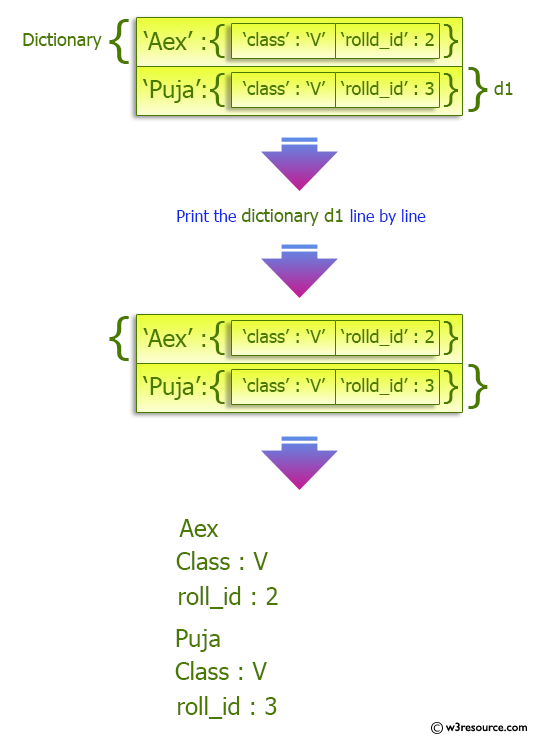﻿ Python: Print a dictionary line by line - w3resource# Python: Print a dictionary line by line

## Python dictionary: Exercise-32 with Solution

Write a Python program to print a dictionary line by line.

Sample Solution:-

Python Code:

``````students = {'Aex':{'class':'V',
'rolld_id':2},
'Puja':{'class':'V',
'roll_id':3}}
for a in students:
print(a)
for b in students[a]:
print (b,':',students[a][b])
```
```

Sample Output:

```Aex
class : V
rolld_id : 2
Puja
class : V
roll_id : 3
```

Pictorial Presentation:## Visualize Python code execution:

The following tool visualize what the computer is doing step-by-step as it executes the said program:

Python Code Editor:

Have another way to solve this solution? Contribute your code (and comments) through Disqus.

What is the difficulty level of this exercise?

Test your Programming skills with w3resource's quiz.

﻿

## Python: Tips of the Day

Decapitalizes the first letter of a string:

Example:

```def tips_decapitalize(s, upper_rest=False):
return s[:1].lower() + (s[1:].upper() if upper_rest else s[1:])
print(tips_decapitalize('PythonTips'))
print(tips_decapitalize('PythonTips', True))
```

Output:

```pythonTips
pYTHONTIPS
```